# Ranking NFL Teams¶

In :
import os
import dateutil
import json
import networkx as nx # read it in with NX
import igraph as ig   # process it in Igraph
import numpy as np
import pandas as pd
import statsmodels.api as sm
import scipy.stats as sps
from sklearn import linear_model
from patsy import dmatrices, dmatrix


## Data¶

In :
!mkdir -p data
if not os.path.exists('data/NFL_Data_Historical.zip'):
!curl -o "data/#1" http://armchairanalysis.com/{NFL_Data_Historical.zip}
if not os.path.exists('data/GAMES.csv'):
!unzip -d data data/NFL_Data_Historical.zip

In :
armchair_games = pd.read_csv('data/GAMES.csv', index_col=0)


The 2012 games are not included, so we'll need to append them. This is kind of a mess because the Pro Football Reference data are weird.

In :
games2012 = pd.read_csv('data/nfl2012.csv')
teams = sorted(armchair_games['H'].unique())
# These just happen to line up except for Seattle/SF
team_names = {name: team for name, team in zip(sorted(games2012['Loser'].unique()), teams)}
team_names['San Francisco 49ers'] = 'SF'
team_names['Seattle Seahawks'] = 'SEA'

games2012['H'] = games2012.apply(lambda x: x['Loser'] if x['Unnamed: 5'] == '@' else x['Winner'] , axis=1)
games2012['V'] = games2012.apply(lambda x: x['Winner'] if x['Unnamed: 5'] == '@' else x['Loser'] , axis=1)
games2012['H'] = games2012['H'].apply(team_names.get)
games2012['V'] = games2012['V'].apply(team_names.get)

games2012['PTSH'] = games2012.apply(lambda x: x['PtsL'] if x['Unnamed: 5'] == '@' else x['PtsW'] , axis=1)
games2012['PTSV'] = games2012.apply(lambda x: x['PtsW'] if x['Unnamed: 5'] == '@' else x['PtsL'] , axis=1)
games2012['SEAS'] = 2012
max_gid = int(armchair_games.index.astype(int).max())
games2012.index = range(max_gid+1, max_gid+1+games2012.shape)
games2012.index.name = 'GID'
games2012 = games2012.rename(columns={'Week': 'WEEK'})[['SEAS', 'WEEK', 'H', 'V', 'PTSH', 'PTSV']]

games = pd.concat([armchair_games, games2012])
games.to_csv('web/all.games.csv')


These utility functions willl transform a Pandas dataframe into either a graph, a matrix, or a set of model matrices for using a Statsmodels/Sklearn supervised model.

In :
teams = sorted(games['H'].unique())
team_ids = {team: i for i, team in enumerate(teams)}

def make_graph(df):
"""Turn a set of games into a graph representation."""
# convert teams to ints for igraph
edges = []
for gid, row in df.iterrows():
if row['PTSH'] > row['PTSV']:
winner = row['H']
loser = row['V']
else:
winner = row['V']
loser = row['H']
edges.append((team_ids[loser], team_ids[winner]))
return ig.Graph(edges=edges, directed=True, vertex_attrs={'name': teams})

def make_matrix(df, elements=lambda x: (1, 0) if x['PTSH'] > x['PTSV'] else (0, 1)):
"""Turn a set of games into a matrix representation."""
k = len(teams)
mat = np.zeros((k, k))
for ix, row in df.iterrows():
a, b = elements(row)
mat[team_ids[row['H']], team_ids[row['V']]] = a
mat[team_ids[row['V']], team_ids[row['H']]] = b
return mat

def make_model_matrix(df):
dummies = {team: (df['H'] == team).astype(np.int) - (df['V'] == team).astype(np.int)
for team in teams}
df2 = pd.DataFrame(dummies)
df2['win'] = (df['PTSH'] > df['PTSV']).astype(np.int)

y, X = dmatrices('win ~ 0 + %s' % ' + '.join(teams), df2)
return y, X


### Getting ESPN's Power Rankings¶

In :
import requests
from BeautifulSoup import BeautifulSoup as Soup

def fetch_power_rankings(year, week):
"""Get the rankings from ESPN for a given year/week."""
url = 'http://espn.go.com/nfl/powerrankings/_/year/%i/week/%i' % (year, week)
page = requests.get(url)
soup = Soup(page.content)
raw = [(int(td.text), td.parent.findAll('a').get('href')) for td in soup.findAll(attrs={'class': 'pr-rank'})]
ranks = {}
for rank, url in raw:
ranks[url.split('/')[-2].upper()] = rank
ranks['WAS'] = ranks.pop('WSH')
return ranks

if not os.path.exists('data/espn.rankings.json'):
espn_rankings = {}
for year in range(2002, 2013):
espn_rankings[year] = {}
for week in (9, 18):
espn_rankings[year][week] = fetch_power_rankings(year, week)

with open('data/espn.rankings.json', 'w') as f:
espn_rankings = json.dump(espn_rankings, f)

else:
with open('data/espn.rankings.json') as f:

def espn_rank(df):
week = df['WEEK'].max()
season = df['SEAS'].max()
return espn_rankings[str(season)][str(week+1)]


We'll make a dataframe for the 2012 season to use as an example.

In :
g12 = games[(games['SEAS'] == 2012)*(games['WEEK'] < 18)]


## Evaluation Methods¶

In :
def rank_to_vector(rank):
return np.array([rank[team] for team in teams])

def zero_one_loss(df, rank):
homebetter = df.H.map(rank.get) < df.V.map(rank.get)
homewin = df.PTSH > df.PTSV
return (homewin * homebetter + (1-homewin)*(1-homebetter)).sum() / float(df.shape)

def misrank_loss(df, rank):
winner_rank = games.Winner.map(rank.get)
loser_rank = games.Loser.map(rank.get)
return ((winner_rank > loser_rank)*(winner_rank - loser_rank)).sum()

def eval_espn_similarity(rank_func, year, week):
week8 = games[(games['SEAS'] == year)*(games['WEEK'] <= week)]
ours = rank_to_vector(rank_func(week8))
theirs = rank_to_vector(espn_rankings[str(year)][str(week+1)])

return sps.kendalltau(ours, theirs)

def eval_description(rank_func, year):
season = games[(games['SEAS'] == year)*(games['WEEK'] < 18)]
rank = rank_func(season)
return zero_one_loss(season, rank)

def eval_in_season_prediction(rank_func, year):
first_half = games[(games['SEAS'] == year)*(games['WEEK'] < 9)]
second_half = games[(games['SEAS'] == year)*(games['WEEK'] < 18)*(games['WEEK'] >= 9)]
rank = rank_func(first_half)
return zero_one_loss(second_half, rank)

def eval_postseason_prediction(rank_func, year):
in_season = games[(games['SEAS'] == year)*(games['WEEK'] < 18)]
post_season = games[(games['SEAS'] == year)*(games['WEEK'] >= 18)]
rank = rank_func(in_season)
return zero_one_loss(post_season, rank)


## Ranking Methods¶

### Wins¶

In :
def wins(df):
winner = df.apply(lambda x: x['H'] if x['PTSH'] > x['PTSV'] else x['V'], axis=1)
w = {team: 31 for team in df['H'].unique()}
for rank, (team, ws) in enumerate(w.iteritems()):
w[team] = rank
return w


### Pythagorean Wins¶

pythagorean wins = $\frac{p^{\beta}}{p^{\beta} + q^{\beta}}$

In :
def pythagorean_wins(df, beta=2.37):
home = df.groupby('H')[['PTSH', 'PTSV']].sum().rename(columns={'PTSH': 'PTSFOR', 'PTSV': 'PTSAG'}, index={'H': 'TEAM'})
away = df.groupby('V')[['PTSH', 'PTSV']].sum().rename(columns={'PTSV': 'PTSFOR', 'PTSH': 'PTSAG'}, index={'V': 'TEAM'})
newdf = pd.concat([home, away]).groupby(level=0).sum()
winrate = newdf['PTSFOR']**beta / (newdf['PTSFOR']**beta + newdf['PTSAG']**beta)
return dict((winrate*-1).rank().astype(int).iteritems())


### Eigenvector Methods¶

Power iteration code comes from The Glowing Python's blogpost.

PageRank with no damping (damping = 1) should be equivalent to taking the largest eigvenvector.

In :
def pagerank(g, damping=1):
values = g.pagerank(damping=damping)
g_pr = {vert['name']: val for vert, val in zip(g.vs, values)}
return g_pr #{key: i for i, (key, v) in enumerate(sorted(g_pr.iteritems(), key=lambda x: x))}

def power_iteration(m, iters=10000, x0=None):
if x0 == None:
x0 = np.ones(m.shape)
x0 /= np.linalg.norm(x0, 1)

for i in range(iters):
x0 = np.dot(m,x0)
x0 /= np.linalg.norm(x0,1)

scores = {team: x0[i] for team, i in team_ids.iteritems()}
return {team: rank for rank, (team, score) in enumerate(sorted(scores.iteritems(), key=lambda x: x, reverse=True))}

def eigen_rank(df):
m = make_matrix(df)
return power_iteration(m)

def eigen_rank_scores(df):
"""Uses points scored instead of dummy variables for wins"""
m = make_matrix(df, elements=lambda x: (x['PTSH'], x['PTSV']))
return power_iteration(m)

def score_transform(s1, s2):
x = (s1 + 1.0) / (s1 + s2 + 2.0)
return 0.5 + 0.5 * np.sign(x - 0.5) * np.sqrt(np.abs(2*x - 1))

def eigen_rank_scores_nonlinear(df):
"""Uses a transformed version of score with gives less credit for blowouts
See Keener (1993).
"""
m = make_matrix(df, elements=lambda x: (score_transform(x['PTSH'], x['PTSV']), score_transform(x['PTSV'], x['PTSH'])))
return power_iteration(m)


In :
def logit_rank(df, c=1.0):
y, X = make_model_matrix(df)
clf = linear_model.LogisticRegression(C=c, penalty='l2', tol=1e-6, fit_intercept=False)
clf.fit(X, y)
return {team: rank for rank, (team, score) in enumerate(sorted(zip(teams, clf.coef_), key=lambda x: x, reverse=True))}


### Optimal Rankings¶

In :
def optimal_rank(df):
g = make_graph(df)
feedback_arcs = g.feedback_arc_set(method='exact')
g.delete_edges(feedback_arcs)
g_sort = g.topological_sorting()
return {g.vs[ix]['name']: i for i, ix in enumerate(reversed(g_sort))}


## Evaluating our Rankings¶

In :
rankers = {
'wins': wins,
'pythagorean wins': pythagorean_wins,
'eigenvector': eigen_rank,
'eigenvector2': eigen_rank_scores_nonlinear,
'btl': logit_rank,
'optimal': optimal_rank,
'espn': espn_rank,
}

evaluations = {
'descriptive': eval_description,
'predict_in_season': eval_in_season_prediction,
'predict_postseason': eval_postseason_prediction,
'espn_similarity8': lambda rank, year: eval_espn_similarity(rank, year, 8),
'espn_similarity17': lambda rank, year: eval_espn_similarity(rank, year, 17)
}

results = []
for name, ranker in rankers.iteritems():
for eval_name, evaluation in evaluations.iteritems():
for year in range(2002, 2013):
metric = evaluation(ranker, year)
results.append({
'rank': name,
'eval': eval_name,
'year': year,
'value': metric,
})

eigenvector2 espn_similarity17 2002
eigenvector2 espn_similarity17 2003
eigenvector2 espn_similarity17 2004
eigenvector2 espn_similarity17 2005
eigenvector2 espn_similarity17 2006
eigenvector2 espn_similarity17 2007
eigenvector2 espn_similarity17 2008
eigenvector2 espn_similarity17 2009
eigenvector2 espn_similarity17 2010
eigenvector2 espn_similarity17 2011
eigenvector2 espn_similarity17 2012
eigenvector2 espn_similarity8 2002
eigenvector2 espn_similarity8 2003
eigenvector2 espn_similarity8 2004
eigenvector2 espn_similarity8 2005
eigenvector2 espn_similarity8 2006
eigenvector2 espn_similarity8 2007
eigenvector2 espn_similarity8 2008
eigenvector2 espn_similarity8 2009
eigenvector2 espn_similarity8 2010
eigenvector2 espn_similarity8 2011
eigenvector2 espn_similarity8 2012
eigenvector2 predict_in_season 2002
eigenvector2 predict_in_season 2003
eigenvector2 predict_in_season 2004
eigenvector2 predict_in_season 2005
eigenvector2 predict_in_season 2006
eigenvector2 predict_in_season 2007
eigenvector2 predict_in_season 2008
eigenvector2 predict_in_season 2009
eigenvector2 predict_in_season 2010
eigenvector2 predict_in_season 2011
eigenvector2 predict_in_season 2012
eigenvector2 descriptive 2002
eigenvector2 descriptive 2003
eigenvector2 descriptive 2004
eigenvector2 descriptive 2005
eigenvector2 descriptive 2006
eigenvector2 descriptive 2007
eigenvector2 descriptive 2008
eigenvector2 descriptive 2009
eigenvector2 descriptive 2010
eigenvector2 descriptive 2011
eigenvector2 descriptive 2012
eigenvector2 predict_postseason 2002
eigenvector2 predict_postseason 2003
eigenvector2 predict_postseason 2004
eigenvector2 predict_postseason 2005
eigenvector2 predict_postseason 2006
eigenvector2 predict_postseason 2007
eigenvector2 predict_postseason 2008
eigenvector2 predict_postseason 2009
eigenvector2 predict_postseason 2010
eigenvector2 predict_postseason 2011
eigenvector2 predict_postseason 2012
espn espn_similarity17 2002
espn espn_similarity17 2003
espn espn_similarity17 2004
espn espn_similarity17 2005
espn espn_similarity17 2006
espn espn_similarity17 2007
espn espn_similarity17 2008
espn espn_similarity17 2009
espn espn_similarity17 2010
espn espn_similarity17 2011
espn espn_similarity17 2012
espn espn_similarity8 2002
espn espn_similarity8 2003
espn espn_similarity8 2004
espn espn_similarity8 2005
espn espn_similarity8 2006
espn espn_similarity8 2007
espn espn_similarity8 2008
espn espn_similarity8 2009
espn espn_similarity8 2010
espn espn_similarity8 2011
espn espn_similarity8 2012
espn predict_in_season 2002
espn predict_in_season 2003
espn predict_in_season 2004
espn predict_in_season 2005
espn predict_in_season 2006
espn predict_in_season 2007
espn predict_in_season 2008
espn predict_in_season 2009
espn predict_in_season 2010
espn predict_in_season 2011
espn predict_in_season 2012
espn descriptive 2002
espn descriptive 2003
espn descriptive 2004
espn descriptive 2005
espn descriptive 2006
espn descriptive 2007
espn descriptive 2008
espn descriptive 2009
espn descriptive 2010
espn descriptive 2011
espn descriptive 2012
espn predict_postseason 2002
espn predict_postseason 2003
espn predict_postseason 2004
espn predict_postseason 2005
espn predict_postseason 2006
espn predict_postseason 2007
espn predict_postseason 2008
espn predict_postseason 2009
espn predict_postseason 2010
espn predict_postseason 2011
espn predict_postseason 2012
wins espn_similarity17 2002
wins espn_similarity17 2003
wins espn_similarity17 2004
wins espn_similarity17 2005
wins espn_similarity17 2006
wins espn_similarity17 2007
wins espn_similarity17 2008
wins espn_similarity17 2009
wins espn_similarity17 2010
wins espn_similarity17 2011
wins espn_similarity17 2012
wins espn_similarity8 2002
wins espn_similarity8 2003
wins espn_similarity8 2004
wins espn_similarity8 2005
wins espn_similarity8 2006
wins espn_similarity8 2007
wins espn_similarity8 2008
wins espn_similarity8 2009
wins espn_similarity8 2010
wins espn_similarity8 2011
wins espn_similarity8 2012
wins predict_in_season 2002
wins predict_in_season 2003
wins predict_in_season 2004
wins predict_in_season 2005
wins predict_in_season 2006
wins predict_in_season 2007
wins predict_in_season 2008
wins predict_in_season 2009
wins predict_in_season 2010
wins predict_in_season 2011
wins predict_in_season 2012
wins descriptive 2002
wins descriptive 2003
wins descriptive 2004
wins descriptive 2005
wins descriptive 2006
wins descriptive 2007
wins descriptive 2008
wins descriptive 2009
wins descriptive 2010
wins descriptive 2011
wins descriptive 2012
wins predict_postseason 2002
wins predict_postseason 2003
wins predict_postseason 2004
wins predict_postseason 2005
wins predict_postseason 2006
wins predict_postseason 2007
wins predict_postseason 2008
wins predict_postseason 2009
wins predict_postseason 2010
wins predict_postseason 2011
wins predict_postseason 2012
optimal espn_similarity17 2002
optimal espn_similarity17 2003
optimal espn_similarity17 2004
optimal espn_similarity17 2005
optimal espn_similarity17 2006
optimal espn_similarity17 2007
optimal espn_similarity17 2008
optimal espn_similarity17 2009
optimal espn_similarity17 2010
optimal espn_similarity17 2011
optimal espn_similarity17 2012
optimal espn_similarity8 2002
optimal espn_similarity8 2003
optimal espn_similarity8 2004
optimal espn_similarity8 2005
optimal espn_similarity8 2006
optimal espn_similarity8 2007
optimal espn_similarity8 2008
optimal espn_similarity8 2009
optimal espn_similarity8 2010
optimal espn_similarity8 2011
optimal espn_similarity8 2012
optimal predict_in_season 2002
optimal predict_in_season 2003
optimal predict_in_season 2004
optimal predict_in_season 2005
optimal predict_in_season 2006
optimal predict_in_season 2007
optimal predict_in_season 2008
optimal predict_in_season 2009
optimal predict_in_season 2010
optimal predict_in_season 2011
optimal predict_in_season 2012
optimal descriptive 2002
optimal descriptive 2003
optimal descriptive 2004
optimal descriptive 2005
optimal descriptive 2006
optimal descriptive 2007
optimal descriptive 2008
optimal descriptive 2009
optimal descriptive 2010
optimal descriptive 2011
optimal descriptive 2012
optimal predict_postseason 2002
optimal predict_postseason 2003
optimal predict_postseason 2004
optimal predict_postseason 2005
optimal predict_postseason 2006
optimal predict_postseason 2007
optimal predict_postseason 2008
optimal predict_postseason 2009
optimal predict_postseason 2010
optimal predict_postseason 2011
optimal predict_postseason 2012
btl espn_similarity17 2002
btl espn_similarity17 2003
btl espn_similarity17 2004
btl espn_similarity17 2005
btl espn_similarity17 2006
btl espn_similarity17 2007
btl espn_similarity17 2008
btl espn_similarity17 2009
btl espn_similarity17 2010
btl espn_similarity17 2011
btl espn_similarity17 2012
btl espn_similarity8 2002
btl espn_similarity8 2003
btl espn_similarity8 2004
btl espn_similarity8 2005
btl espn_similarity8 2006
btl espn_similarity8 2007
btl espn_similarity8 2008
btl espn_similarity8 2009
btl espn_similarity8 2010
btl espn_similarity8 2011
btl espn_similarity8 2012
btl predict_in_season 2002
btl predict_in_season 2003
btl predict_in_season 2004
btl predict_in_season 2005
btl predict_in_season 2006
btl predict_in_season 2007
btl predict_in_season 2008
btl predict_in_season 2009
btl predict_in_season 2010
btl predict_in_season 2011
btl predict_in_season 2012
btl descriptive 2002
btl descriptive 2003
btl descriptive 2004
btl descriptive 2005
btl descriptive 2006
btl descriptive 2007
btl descriptive 2008
btl descriptive 2009
btl descriptive 2010
btl descriptive 2011
btl descriptive 2012
btl predict_postseason 2002
btl predict_postseason 2003
btl predict_postseason 2004
btl predict_postseason 2005
btl predict_postseason 2006
btl predict_postseason 2007
btl predict_postseason 2008
btl predict_postseason 2009
btl predict_postseason 2010
btl predict_postseason 2011
btl predict_postseason 2012
pythagorean wins espn_similarity17 2002
pythagorean wins espn_similarity17 2003
pythagorean wins espn_similarity17 2004
pythagorean wins espn_similarity17 2005
pythagorean wins espn_similarity17 2006
pythagorean wins espn_similarity17 2007
pythagorean wins espn_similarity17 2008
pythagorean wins espn_similarity17 2009
pythagorean wins espn_similarity17 2010
pythagorean wins espn_similarity17 2011
pythagorean wins espn_similarity17 2012
pythagorean wins espn_similarity8 2002
pythagorean wins espn_similarity8 2003
pythagorean wins espn_similarity8 2004
pythagorean wins espn_similarity8 2005
pythagorean wins espn_similarity8 2006
pythagorean wins espn_similarity8 2007
pythagorean wins espn_similarity8 2008
pythagorean wins espn_similarity8 2009
pythagorean wins espn_similarity8 2010
pythagorean wins espn_similarity8 2011
pythagorean wins espn_similarity8 2012
pythagorean wins predict_in_season 2002
pythagorean wins predict_in_season 2003
pythagorean wins predict_in_season 2004
pythagorean wins predict_in_season 2005
pythagorean wins predict_in_season 2006
pythagorean wins predict_in_season 2007
pythagorean wins predict_in_season 2008
pythagorean wins predict_in_season 2009
pythagorean wins predict_in_season 2010
pythagorean wins predict_in_season 2011
pythagorean wins predict_in_season 2012
pythagorean wins descriptive 2002
pythagorean wins descriptive 2003
pythagorean wins descriptive 2004
pythagorean wins descriptive 2005
pythagorean wins descriptive 2006
pythagorean wins descriptive 2007
pythagorean wins descriptive 2008
pythagorean wins descriptive 2009
pythagorean wins descriptive 2010
pythagorean wins descriptive 2011
pythagorean wins descriptive 2012
pythagorean wins predict_postseason 2002
pythagorean wins predict_postseason 2003
pythagorean wins predict_postseason 2004
pythagorean wins predict_postseason 2005
pythagorean wins predict_postseason 2006
pythagorean wins predict_postseason 2007
pythagorean wins predict_postseason 2008
pythagorean wins predict_postseason 2009
pythagorean wins predict_postseason 2010
pythagorean wins predict_postseason 2011
pythagorean wins predict_postseason 2012
eigenvector espn_similarity17 2002
eigenvector espn_similarity17 2003
eigenvector espn_similarity17 2004
eigenvector espn_similarity17 2005
eigenvector espn_similarity17 2006
eigenvector espn_similarity17 2007
eigenvector espn_similarity17 2008
eigenvector espn_similarity17 2009
eigenvector espn_similarity17 2010
eigenvector espn_similarity17 2011
eigenvector espn_similarity17 2012
eigenvector espn_similarity8 2002
eigenvector espn_similarity8 2003
eigenvector espn_similarity8 2004
eigenvector espn_similarity8 2005
eigenvector espn_similarity8 2006
eigenvector espn_similarity8 2007
eigenvector espn_similarity8 2008
eigenvector espn_similarity8 2009
eigenvector espn_similarity8 2010
eigenvector espn_similarity8 2011
eigenvector espn_similarity8 2012
eigenvector predict_in_season 2002
eigenvector predict_in_season 2003
eigenvector predict_in_season 2004
eigenvector predict_in_season 2005
eigenvector predict_in_season 2006
eigenvector predict_in_season 2007
eigenvector predict_in_season 2008
eigenvector predict_in_season 2009
eigenvector predict_in_season 2010
eigenvector predict_in_season 2011
eigenvector predict_in_season 2012
eigenvector descriptive 2002
eigenvector descriptive 2003
eigenvector descriptive 2004
eigenvector descriptive 2005
eigenvector descriptive 2006
eigenvector descriptive 2007
eigenvector descriptive 2008
eigenvector descriptive 2009
eigenvector descriptive 2010
eigenvector descriptive 2011
eigenvector descriptive 2012
eigenvector predict_postseason 2002
eigenvector predict_postseason 2003
eigenvector predict_postseason 2004
eigenvector predict_postseason 2005
eigenvector predict_postseason 2006
eigenvector predict_postseason 2007
eigenvector predict_postseason 2008
eigenvector predict_postseason 2009
eigenvector predict_postseason 2010
eigenvector predict_postseason 2011
eigenvector predict_postseason 2012

In :
resdf = pd.DataFrame(results)
resdf.set_index(['eval', 'year', 'rank'], inplace=True)

Out:
<class 'pandas.core.frame.DataFrame'>
MultiIndex: 385 entries, (espn_similarity17, 2002, eigenvector2) to (predict_postseason, 2012, eigenvector)
Data columns:
value    385  non-null values
dtypes: float64(1)

## Making Rankings¶

In :
results2 = []
for name, ranker in rankers.iteritems():
for year in range(2002, 2013):
df = games[(games.SEAS == year)*(games.WEEK < 18)]
rank = ranker(df)
for rank, (team, value) in enumerate(sorted(rank.iteritems(), key=lambda x: x)):
results2.append({
'method': name,
'year': year,
'rank': rank+1
})
all_rankings = pd.DataFrame(results2)
all_rankings.to_csv('web/all.rankings.csv')

In :
all_rankings.method.unique()

Out:
array([eigenvector2, espn, wins, optimal, btl, pythagorean wins,
eigenvector], dtype=object)
In :
figsize(12, 8)
fig = resdf.ix['descriptive'].unstack()['value'].plot()
savefig('web/slides/img/desc.perf.png')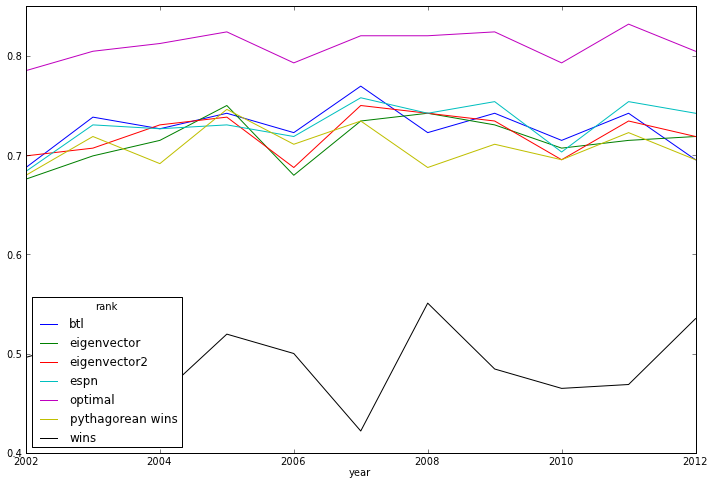In :
fig = resdf.ix['espn_similarity8'].unstack()['value'].plot()
savefig('web/slides/img/espn8.perf.png')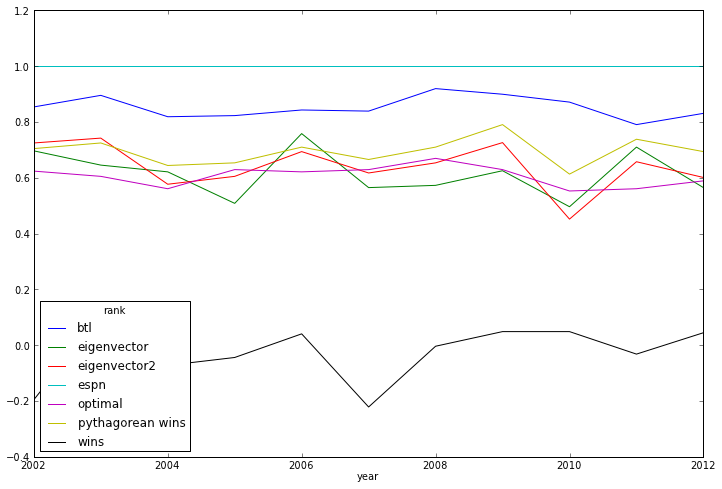In :
fig = resdf.ix['espn_similarity17'].unstack()['value'].plot()
savefig('web/slides/img/espn17.perf.png')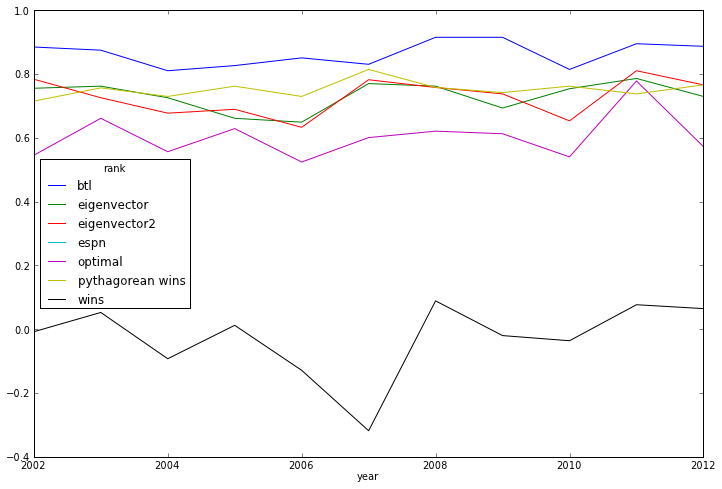In :
fig = resdf.ix['predict_in_season'].unstack()['value'].plot()
savefig('web/slides/img/season.perf.png')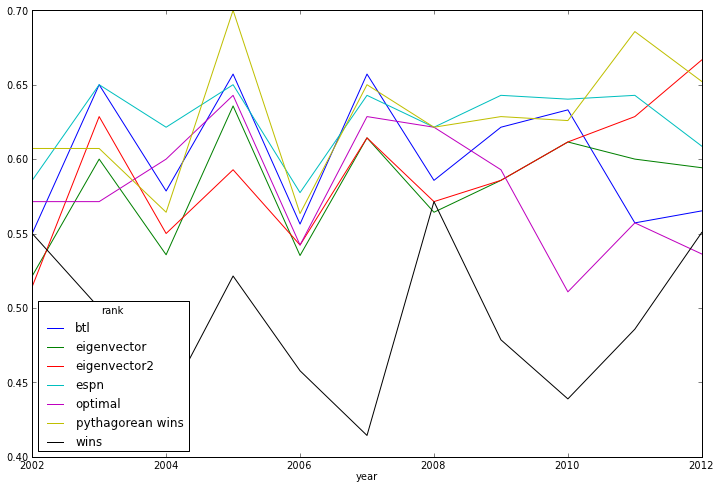In :
fig = resdf.ix['predict_postseason'].unstack()['value'].plot()
savefig('web/slides/img/postseason.perf.png')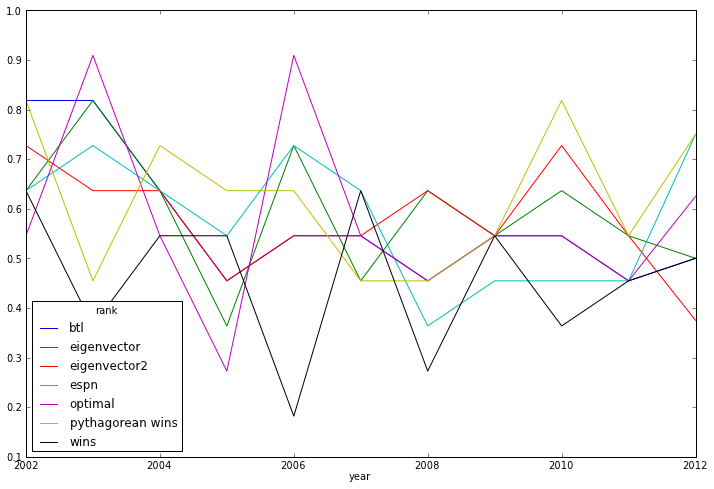## 2012 Ranking Tables¶

In :
def emit_table(rank, fn):
ranked = [t for t, r in sorted(rank.iteritems(), key=lambda x: x)]
with open(fn, 'w') as f:
f.write('<table class="ranking-table" style="margin-left: auto; margin-right: auto;">')

for i in range(8):
f.write('<tr>')
for j in range(4):
ix = i + j*8
f.write('<td>%i</td><td><img src="../logos/%s.png" width=70 height=35 style="border:none;"/></td>' % (ix+1, ranked[ix]))
f.write('</tr>')
f.write('</table>')

In :
emit_table(pythagorean_wins(g12), 'web/slides/pythagorean.html')
emit_table(eigen_rank_scores_nonlinear(g12), 'web/slides/eigenvector.html')
emit_table(logit_rank(g12), 'web/slides/btl.html')
emit_table(optimal_rank(g12), 'web/slides/optimal.html')


## NFC East Matrix Example¶

In :
n = A.shape
w,v = np.linalg.eig(A)
abs(np.real(v[:n,0]) / np.linalg.norm(v[:n,0],1))

A = np.matrix("[0 1 2 0; 1 0 1 1; 0 1 0 0; 2 1 2 0]")
mat = A.copy()
for i in range(100):
mat = mat.dot(A)
print mat.dot(np.ones(4))

[[  7.35615860e+18  -1.12086486e+19   7.02600656e+18  -1.08784965e+19]]